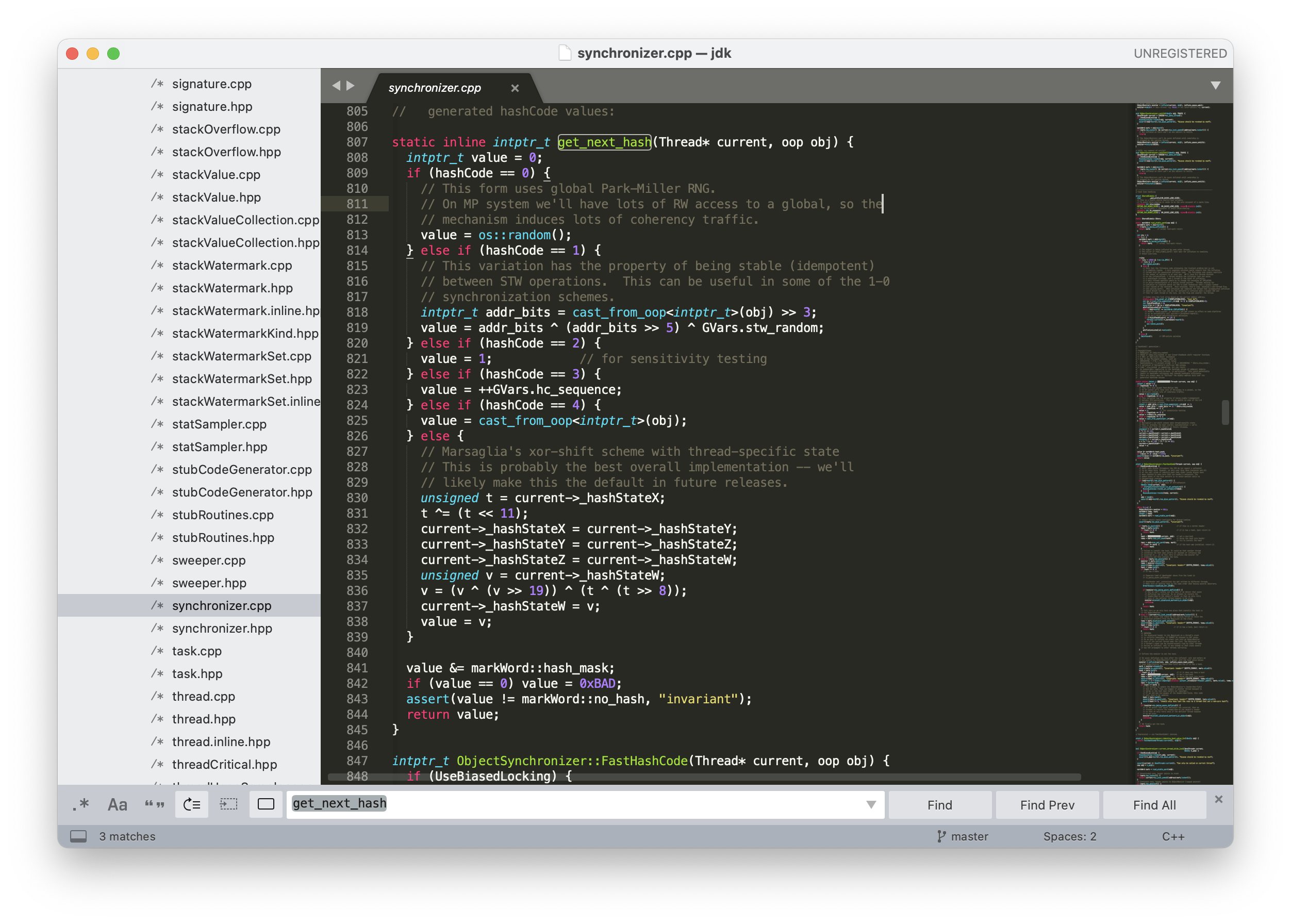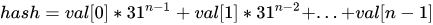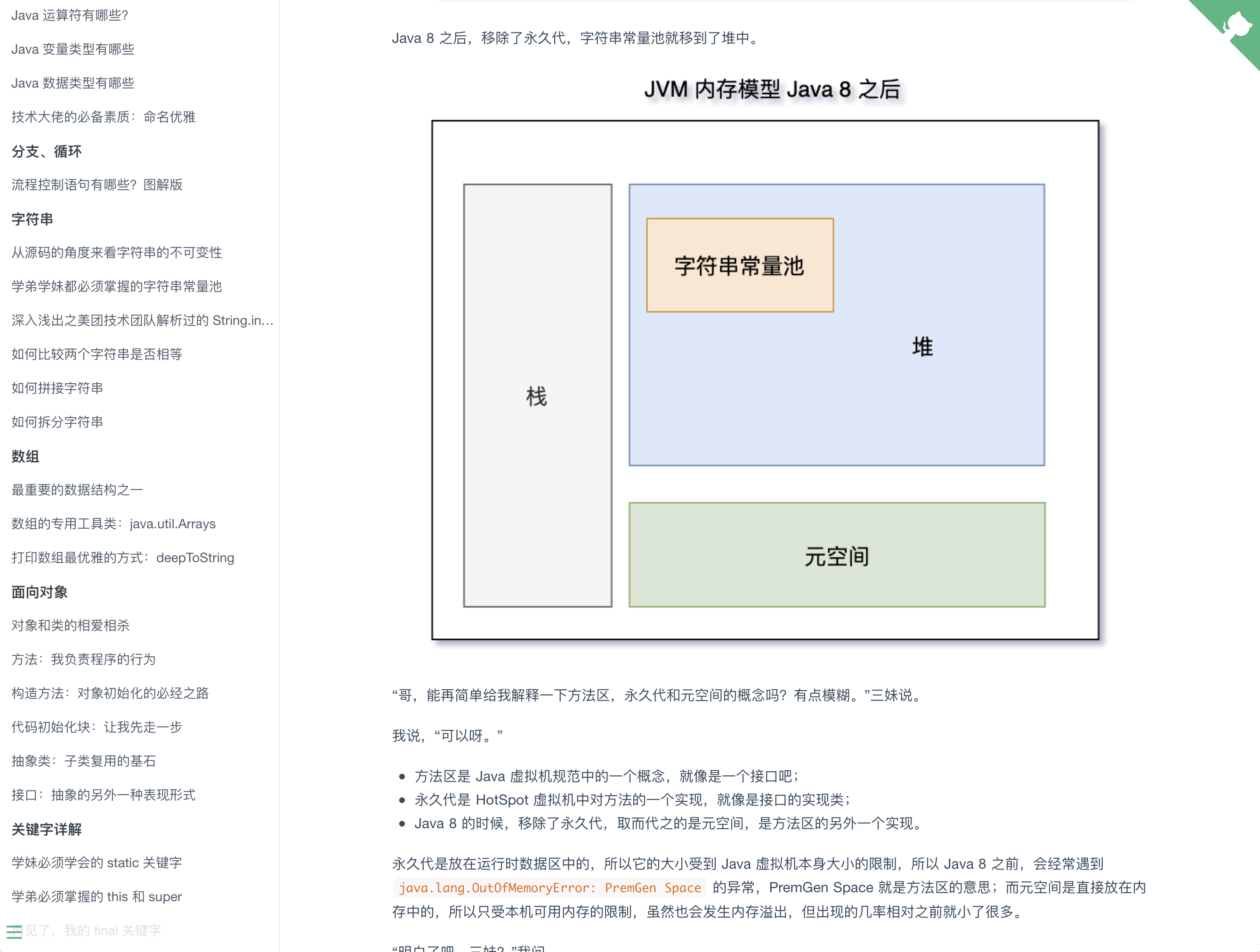# 为了彻底搞懂 hashCode，我连 JDK 的源码都没放过

2021/08/10

Object 类中就包含了 `hashCode()` 方法：

``````@HotSpotIntrinsicCandidate
public native int hashCode();
``````GitHub 开源地址（欢迎 star）：https://github.com/itwanger/jmx-java

• 获取对象的哈希值；
• 和之前已经存在的哈希值进行比较，如果不相等，直接存进去；
• 如果有相等的，再调用 `equals()` 方法进行对象之间的比较，如果相等，不存了；
• 如果不等，说明哈希冲突了，增加一个链表，存放新的对象；
• 如果链表的长度大于 8，转为红黑树来处理。

``````static final int hash(Object key) {
int h;
return (key == null) ? 0 : (h = key.hashCode()) ^ (h >>> 16);
}
``````

``````public int hashCode() {
int h = hash;
if (h == 0 && value.length > 0) {
hash = h = isLatin1() ? StringLatin1.hashCode(value)
: StringUTF16.hashCode(value);
}
return h;
}
public static int hashCode(byte[] value) {
int h = 0;
int length = value.length >> 1;
for (int i = 0; i < length; i++) {
h = 31 * h + getChar(value, i);
}
return h;
}
``````

• 如果两个对象调用 `equals()` 方法得到的结果为 true，调用 `hashCode()` 方法得到的结果必定相等；
• 如果两个对象调用 `hashCode()` 方法得到的结果不相等，调用 `equals()` 方法得到的结果必定为 false；

• 如果两个对象调用 `equals()` 方法得到的结果为 false，调用 `hashCode()` 方法得到的结果不一定不相等；
• 如果两个对象调用 `hashCode()` 方法得到的结果相等，调用 `equals()` 方法得到的结果不一定为 true；

``````public class Test {
public static void main(String[] args) {
Student s1 = new Student(18, "张三");
Map<Student, Integer> scores = new HashMap<>();
scores.put(s1, 98);
System.out.println(scores.get(new Student(18, "张三")));
}
}
class Student {
private int age;
private String name;

public Student(int age, String name) {
this.age = age;
this.name = name;
}

@Override
public boolean equals(Object o) {
Student student = (Student) o;
return age == student.age &&
Objects.equals(name, student.name);
}
}
``````

`main()` 方法中，18 岁的张三考试得了 98 分，很不错的成绩，我们把张三和成绩放到了 HashMap 中，然后准备输出张三的成绩：

``````null
``````

HashMap 的 `get()` 方法会调用 `hash(key.hashCode())` 计算对象的哈希值，虽然两个不同的 `hashCode()` 结果经过 `hash()` 方法计算后有可能得到相同的结果，但这种概率微乎其微，所以就导致 `scores.get(new Student(18, "张三"))` 无法得到预期的值 18。

`````` @Override
public int hashCode() {
return Objects.hash(age, name);
}
``````

Objects 类的 `hash()` 方法可以针对不同数量的参数生成新的 `hashCode()` 值。

``````public static int hashCode(Object a[]) {
if (a == null)
return 0;

int result = 1;

for (Object element : a)
result = 31 * result + (element == null ? 0 : element.hashCode());

return result;
}
``````《Java 编程思想》这本圣经中有一段话，对 `hashCode()` 方法进行了一段描述。

``````static inline intptr_t get_next_hash(Thread* current, oop obj) {
intptr_t value = 0;
if (hashCode == 0) {
// This form uses global Park-Miller RNG.
// On MP system we'll have lots of RW access to a global, so the
// mechanism induces lots of coherency traffic.
value = os::random();
} else if (hashCode == 1) {
// This variation has the property of being stable (idempotent)
// between STW operations.  This can be useful in some of the 1-0
// synchronization schemes.
intptr_t addr_bits = cast_from_oop<intptr_t>(obj) >> 3;
} else if (hashCode == 2) {
value = 1;            // for sensitivity testing
} else if (hashCode == 3) {
value = ++GVars.hc_sequence;
} else if (hashCode == 4) {
value = cast_from_oop<intptr_t>(obj);
} else {
// Marsaglia's xor-shift scheme with thread-specific state
// This is probably the best overall implementation -- we'll
// likely make this the default in future releases.
unsigned t = current->_hashStateX;
t ^= (t << 11);
current->_hashStateX = current->_hashStateY;
current->_hashStateY = current->_hashStateZ;
current->_hashStateZ = current->_hashStateW;
unsigned v = current->_hashStateW;
v = (v ^ (v >> 19)) ^ (t ^ (t >> 8));
current->_hashStateW = v;
value = v;
}

if (value == 0) value = 0xBAD;
assert(value != markWord::no_hash, "invariant");
return value;
}
``````

• `hashCode==0`，调用操作系统 OS 的 `random()` 方法返回随机数。
• `hashCode == 1`，在 STW（stop-the-world）操作中，这种策略通常用于同步方案中。利用对象地址进行计算，使用不经常更新的随机数（`GVars.stw_random`）参与其中。
• `hashCode == 2`，使用返回 1，用于某些情况下的测试。
• `hashCode == 3`，从 0 开始计算哈希值，不是线程安全的，多个线程可能会得到相同的哈希值。
• `hashCode == 4`，与创建对象的内存位置有关，原样输出。
• `hashCode == 5`，默认值，支持多线程，使用了 Marsaglia 的 xor-shift 算法产生伪随机数。所谓的 xor-shift 算法，简单来说，看起来就是一个移位寄存器，每次移入的位由寄存器中若干位取异或生成。所谓的伪随机数，不是完全随机的，但是真随机生成比较困难，所以只要能通过一定的随机数统计检测，就可以当作真随机数来使用。

### 叨逼叨GitHub 开源地址（欢迎 star）：https://github.com/itwanger/jmx-java

（转载本站文章请注明作者和出处 沉默王二